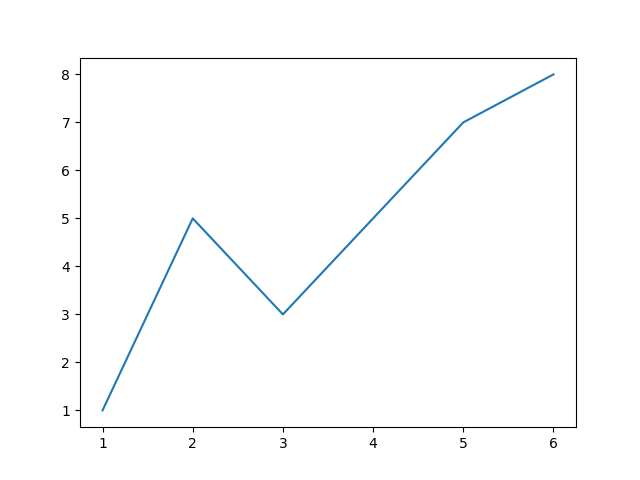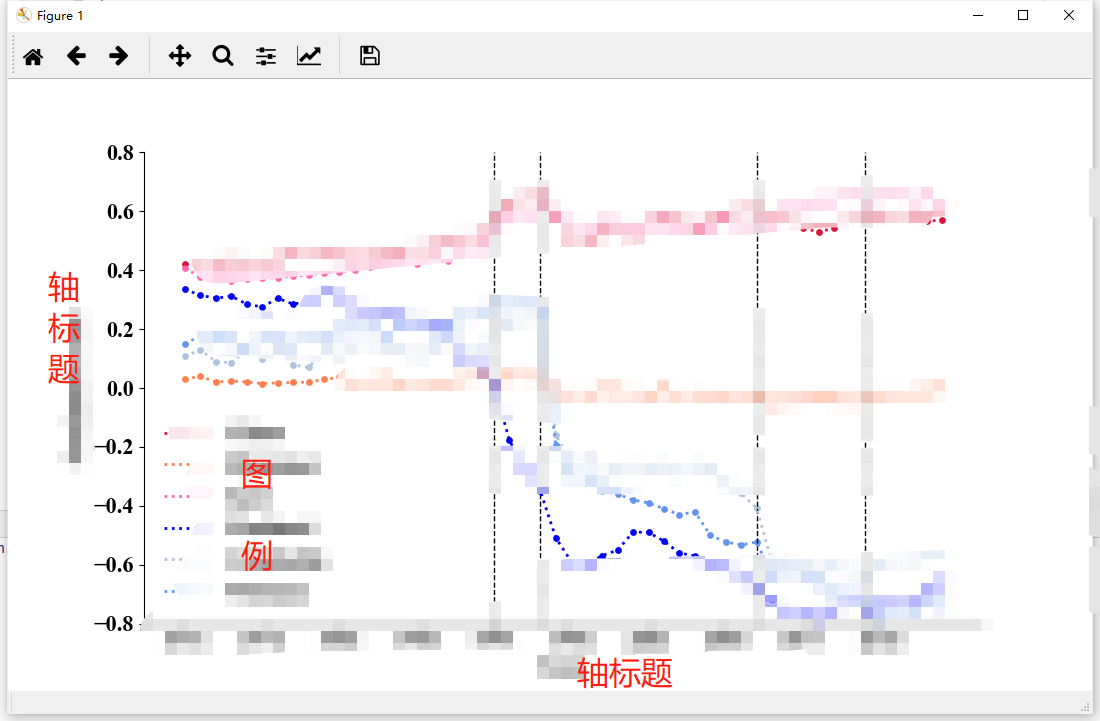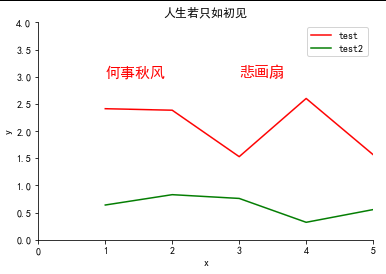• 2022-01-16 04:32:42

Matplotlib 是一个用 Python 编写的流行的数据可视化库。数据可视化有助于以简单直观的方式呈现大型和复杂的数据集。

import matplotlib.pyplot as plt

x = [1, 2, 3, 4, 5, 6]
y = [1, 5, 3, 5, 7, 8]

plt.plot(x, y)
plt.show()

得到一个简单的折线图：​​​​​​​​​​​​​​

更多相关内容
• 这篇文章主要介绍了Python散点图折线图绘制过程解析,文中通过示例代码介绍的非常详细，对大家的学习或者工作具有一定的参考学习价值,需要的朋友可以参考下 在数据分析的过程中，经常需要将数据可视化，目前常使用...
• 接续 【Python_绘图】折线图https://mp-new.csdn.net/mp_blog/creation/editor/117462761

接续博文 “【Python_绘图】折线图” CSDN

# 功能

• 数据：读取表格（可指定Sheet表……也可指定Sheet表中的特定列）
• 绘图样式：多条折线图+散点图 → 标记点折线图
• 设置参数：设置轴标题、添加分界线等

# 代码

import matplotlib.pyplot as plt
import xlrd
import numpy as np
import seaborn as sns

# 设置字体格式，以显示中文
plt.rcParams['font.sans-serif'] = ['Times New Roman']

x_data = []
y1_data = []
y2_data = []
y3_data = []
y4_data = []
y5_data = []
y6_data = []

# 读取表格数据
data = xlrd.open_workbook(r'D:\mekong_NLP\作图\20210512 作图数据汇总\图三 情感折线与词云\20210606 情感分值作图表.xlsx')
table = data.sheets()  # 表格中的Sheet1
x_data = list(range(1971,2021,1))
x_ticks=np.arange(1971,2021,5)

#读取第x行
cap1 = table.row_values(0)
cap2 = table.row_values(1)
cap3 = table.row_values(2)
cap4 = table.row_values(3)
cap5 = table.row_values(4)
cap6 = table.row_values(5)

for i in range(0, len(x_data)):
y1_data.append(cap1[i])
for i in range(0, len(x_data)):
y2_data.append(cap2[i])
for i in range(0, len(x_data)):
y3_data.append(cap3[i])
for i in range(0, len(x_data)):
y4_data.append(cap4[i])
for i in range(0, len(x_data)):
y5_data.append(cap5[i])
for i in range(0, len(x_data)):
y6_data.append(cap6[i])

#逐条 作折线图
plt.plot(x_data, y1_data, c='crimson',linestyle=':',linewidth=2,label='China')
plt.plot(x_data, y2_data, c='coral',linestyle=':',linewidth=2,label='Myanmar')
plt.plot(x_data, y3_data, c='hotpink',linestyle=':',linewidth=2,label='Laos')
plt.plot(x_data, y4_data, c='blue',linestyle=':',linewidth=2,label='Thailand')
plt.plot(x_data, y5_data, c='lightsteelblue',linestyle=':',linewidth=2,label='Cambodia')
plt.plot(x_data, y6_data, c='cornflowerblue',linestyle=':',linewidth=2,label='Vietnam')
#逐条线 作散点图
plt.scatter(x_data, y1_data, c='crimson',s=15)
plt.scatter(x_data, y2_data, c='coral',s=15)
plt.scatter(x_data, y3_data, c='hotpink',s=15)
plt.scatter(x_data, y4_data, c='blue',s=15)
plt.scatter(x_data, y5_data, c='lightsteelblue',s=15)
plt.scatter(x_data, y6_data, c='cornflowerblue',s=15)

#修改图 相关参数
#plt.title('F1值随迭代次数的变化')
plt.xticks(x_ticks)
plt.tick_params(labelsize=16)#横纵标签大小
plt.xlabel('Year',size=16)
plt.ylabel('Sentiment score',size=16)
plt.ylim(ymin=-0.80, ymax=0.80)
plt.vlines(1991, -0.8, 0.8, colors="black", linestyles="dashed",linewidth=1)#分界线
plt.vlines(1994, -0.8, 0.8, colors="black", linestyles="dashed",linewidth=1)
plt.vlines(2008, -0.8, 0.8, colors="black", linestyles="dashed",linewidth=1)
plt.vlines(2015, -0.8, 0.8, colors="black", linestyles="dashed",linewidth=1)

leg=plt.legend(columnspacing=1,fontsize=16)#图例
leg.get_frame().set_linewidth(0.0)#不显示右边与上边线
sns.despine()#不显示右边与上边线
plt.show()#绘图

# 效果示例# 附：使线贴合纵轴

plt.xlim(1971,2021)

展开全文• 主要为大家详细介绍了python散点图实例之随机漫步，具有一定的参考价值，感兴趣的小伙伴们可以参考一下
• 主要为大家详细介绍了python使用matplotlib模块绘制多条折线图散点图的方法，具有一定的参考价值，感兴趣的小伙伴们可以参考一下
• 广告关闭腾讯云11.11云上盛惠 ，精选热门产品助力... 代码创建了x和y之间的散点图，我需要一段代码来过度绘制一条最适合散点图中的数据的行，而且我没有一个内置的pylab函数对我有用。 from matplotlib import *from...广告关闭

腾讯云11.11云上盛惠 ，精选热门产品助力上云，云服务器首年88元起，买的越多返的越多，最高返5000元！

下面是我在文本文件中绘制数据散点图的代码。 我打开的文件包含两列。 左列为x坐标，右列为y坐标。 代码创建了x和y之间的散点图，我需要一段代码来过度绘制一条最适合散点图中的数据的行，而且我没有一个内置的pylab函数对我有用。 from matplotlib import *from pylab import * with open(file.txt) as f:data = out...

引言上期的推文python-matplotlib 学术型散点图绘制 推出后，很多小伙伴比较喜欢? 希望能够推出更多的类似绘制教程推文，当然，也提出了一些问题，比如 学术图表的字体设置、相关性散点图绘制线的完善，以及多图绘制等问题，本期推文就针对此问题进行一一解决。 02. 绘制上下误差线学术性相关性散点图还需添加拟合...导读：什么是散点图？ 可以用来呈现哪些数据关系？ 在数据分析过程中可以解决哪些问题？ 怎样用python绘制散点图？ 本文逐一为你解答。 作者：屈希峰来源：大数据dt（id：bigdatadt）01 概述散点图（scatter）又称散点分布图，是以一个变量为横坐标，另一个变量为纵坐标，利用散点（坐标点）的分布形态反映变量统计...模型的整体拟合优度为 0.357 。 抽象出来，实证过程大致为：导入数据、概览数据、描述性统计、相关系数、绘制散点图回归和模型评估与解释。 接下来，将在 ...也从没有听过描述符（descriptor），那么估计你也不会去搜索它们，然后就永远失去了使用这些 python 独有特性的机会。 （《流畅的 python 》）读到这句话...

作者：zsx_yiyiyi编辑：python大本营阅读文本大概需要 6.66 分钟。 50个matplotlib图的汇编，在数据分析和可视化中最有用。 此列表允许您使用python的matplotlib和seaborn库选择要显示的可视化对象。 1. 关联散点图带边界的气泡图带线性回归最佳拟合线的散点图抖动图计数图边缘直方图边缘箱形图相关图矩阵图2. 偏差...

比较，scatter比plot适合画散点图。 7、 pandas是一个为解决python数据分析而用的包，可以快速构建数据结构。 8、scikit-learn简称sklearn,在导入数据包时只能使用import sklearn。 线性回归函数采用最小二乘函数拟合。 给定n个参数及其对应的x值以及应该输出的y。 训练相关参数的值，再用这个参数给出线性方程预测...

具有拟合线性回归模型的散点图的示例分类 - 识别对象所属的类别。 一个例子是将电子邮件分类为垃圾邮件或合法邮件，或者查看某人的信用评分并批准或拒绝...本指南将提供一个示例填充的使用python的数据挖掘简介，python是最广泛使用的数据挖掘工具之一 - 从清理和数据组织到应用机器学习算法。 首先，让我们更好...

csdn博客作者：zsx_yiyiyi编辑：python大本营50个matplotlib图的汇编，在数据分析和可视化中最有用。 此列表允许您使用python的matplotlib和seaborn库选择要显示的可视化对象。 1. 关联散点图带边界的气泡图带线性回归最佳拟合线的散点图抖动图计数图边缘直方图边缘箱形图相关图矩阵图2. 偏差发散型条形图发散型文本...改变两列数据的顺序，不会对相关系数，和散点图（拟合的函数曲线）造成影响； 对两列数据进行归一化处理，标准化处理，不会影响相关系数； 我们计算的相关系数是线性相关系数，只能反映两者是否具备线性关系。 相关系数高是线性模型拟合程度高的前提； 此外相关系数反映两个变量之间的相关性，多个变量之间的相关性...表明：标准图是一条实线曲线，每个轴都会自动缩放来适应坐标值，但也可以手动进行设置。 多次调用plot函数将会在同一窗口中叠加绘图。 (2)python3.0曲线...或者plot(y)是plot（range(len(y)),y）的简写。 例1：python实现使用200个采样点来绘制sin(x)，并且每隔四个点的位置设置标记。 import numpy as npimport...data.drop(, axis=1)好吧数据很干净，可以进行线性回归！ 简单线性回归造型对于简单的线性回归，只考虑电视广告对销售的影响。 在直接进入建模之前，看一下数据的样子。 使用matplotlib 一个流行的python绘图库来制作散点图。 plt.figure(figsize=(16, 8))plt.scatter( data, data, c=black)plt.xlabel(moneyspent on...

什么是线性回归呢如果我们试图捕捉可以最优解释y观测值的x变量和y变量之间的数学关系我们将在x的观测值形成的散点图中去拟合一条线，那么这条线...大数据文摘作品编译：小明同学君、吴双、yawei xia新年总是跟黄金密不可分。 新年第一天，让我们尝试用python搭建一个机器学习线性回归模型，预测金价！...本篇教程，内容十分丰富，虽然是单篇，大家务必多多练习，可以充当一周的学习内容，静下心来慢慢吸收。 温馨提示1、本节内容重点内容较多， 务必紧跟红色标记。 2、测试数据及代码 见文末客服小姐姐二维码。? 散点图-拟合曲线散点图可用来描述两个连续型变量间的关系。 这里以r中自带的mtcars数据为例绘制第一个示例...这个解释看起来好像不太直白，我是这么理解的：数据拟合就是想办法给一堆散点画一条函数曲线。 至于这条曲线怎么画的问题大家有兴趣的可以去搜索一下，的确不简单，但是电脑这种东西太强大了，我们想要做这个还是非常简单的！ 接下来给大家介excelrpython下的三种实现方法，为便于展示，首先给到一个示例数据——国家...所以行哥分享matplotlib和seaborn速查表给读者们在画图的时候使用，需要pdf版本的话可以在行哥免费的星球里下载? matplotlib速查表? seaborn速查表还有读者反应，有时候在数据可视化的时候不知道使用什么图来展示数据，这里提供了top50的可视化案例进行选择0 初始配置1. 相关性1.1 散点图1.2 气泡图1.3 散点图与最佳...

画散点图创建一个datatemp的文件夹，在其中分别创建data、demo文件夹用于存放数据文件、python程序文件。 把前面图中的数据导入excel中，命名为：“蒸汽...模型结果曲线当然，我们还可以通过判定系数来看一下我们的回归方程与数据拟合的效果好坏，这个在后续的文章中再说...

github.com2seriesmachine-learning-with-pythonblobmasterregressionregression-goodness%20of%20fit.ipynb用于检查线性的自变量散点图可以通过直观检查...残差与预测变量图拟合与残差图归一化残差的直方图qq归一化残差图残差的shapiro-wilk正态检验库克残差距离图预测特征的方差膨胀因子（vif）scikit-learn的...作者：zsx_yiyiyi来源：python大本营50个matplotlib图的汇编，在数据分析和可视化中最有用。 此列表允许您使用python的matplotlib和seaborn库选择要显示的可视化对象。 1. 关联散点图带边界的气泡图带线性回归最佳拟合线的散点图抖动图计数图边缘直方图边缘箱形图相关图矩阵图2. 偏差发散型条形图发散型文本发散型包...

作者：zsx_yiyiyi编辑：python大本营50个matplotlib图的汇编，在数据分析和可视化中最有用。 此列表允许您使用python的matplotlib和seaborn库选择要显示的可视化对象。 1. 关联散点图带边界的气泡图带线性回归最佳拟合线的散点图抖动图计数图边缘直方图边缘箱形图相关图矩阵图2. 偏差发散型条形图发散型文本发散型包...

展开全文• 今天小编就为大家分享一篇python绘制散点图并标记序号的方法，具有很好的参考价值，希望对大家有所帮助。一起跟随小编过来看看吧
• ## python画散点图、折线图

万次阅读 多人点赞 2020-12-10 22:54:24
Python-画图（散点图scatter、保存savefig）及颜色大全 import numpy as np import matplotlib.pyplot as plt plt.rcParams['font.sans-serif']=['SimHei'] plt.rcParams['axes.unicode_minus'] = False #...

## 一、散点图

import numpy as np
import matplotlib.pyplot as plt

plt.rcParams['font.sans-serif']=['SimHei']
plt.rcParams['axes.unicode_minus'] = False
#matplotlib画图中中文显示会有问题，需要这两行设置默认字体

plt.xlabel('x')
#plt.ylabel('')
plt.xlim(xmax=10,xmin=0)
plt.ylim(ymax=2,ymin=0)
x1=[]#自定义点
y1 = []#自定义点
x2=[]#自定义点
y2=[]#自定义点

colors1 = 'r' #点的颜色
colors2 = 'g'
area = np.pi * 4**2  # 点面积
plt.scatter(x1, y1, s=area, c=colors1, alpha=0.4, label='a')
plt.scatter(x2, y2, s=area, c=colors2, alpha=0.4, label='b')
#plt.plot([0,9.5],[9.5,0],linewidth = '0.5',color='#000000')
plt.legend()
plt.yticks(())
plt.title('test')
plt.show()


## 二、折线图

去掉上、右坐标线

import numpy as np
import matplotlib.pyplot as plt
fig, ax = plt.subplots()
ax.spines['right'].set_visible(False)
ax.spines['top'].set_visible(False)


解决中文乱码

plt.rcParams['font.sans-serif']=['SimHei']
plt.rcParams['axes.unicode_minus'] = False


设置坐标轴标题

plt.xlabel('x')
plt.ylabel('y')


设置坐标范围

plt.xlim(xmax=5,xmin=0)
plt.ylim(ymax=4,ymin=0)


自定义坐标描点连线数据

x1=[1,2,3,4,5]
y1=[2.4118,2.3837,1.5294,2.6,1.5663]
x2=x1;
y2=[0.64,0.83034,0.76093,0.32199,0.55581]


开始作图

plt.plot(x1,y1,color='r',label='test')
plt.plot(x2,y2,color='g',label='test2')


设置标题

plt.title(r'人生若只如初见')


添加图标

plt.legend()


在指定坐标处添加文字内容

plt.text(1, 3.0, r'何事秋风',color='r',fontsize=15)
plt.text(3, 3.0, r'悲画扇',color='r',fontsize=15)


整体效果完整代码

import numpy as np
import matplotlib.pyplot as plt
fig, ax = plt.subplots()
ax.spines['right'].set_visible(False)
ax.spines['top'].set_visible(False)
plt.rcParams['font.sans-serif']=['SimHei']
plt.rcParams['axes.unicode_minus'] = False
plt.xlabel('x')
plt.ylabel('y')
plt.xlim(xmax=5,xmin=0)
plt.ylim(ymax=4,ymin=0)
x1=[1,2,3,4,5]
y1=[2.4118,2.3837,1.5294,2.6,1.5663]
x2=x1;
y2=[0.64,0.83034,0.76093,0.32199,0.55581]
plt.plot(x1,y1,color='r',label='test')
plt.plot(x2,y2,color='g',label='test2')
plt.title(r'人生若只如初见')
plt.legend()
plt.text(1, 3.0, r'何事秋风',color='r',fontsize=15)
plt.text(3, 3.0, r'悲画扇',color='r',fontsize=15)


用Pycharm运行不出图

plt.show()


保存图片到本地

其中dpi为图像的清晰度，可改高一点 比如600，图片会更大更清晰

plt.savefig('图片名字.png', dpi=300, bbox_inches='tight')#指定分辨率


## 其他

python plt画图横纵坐标0点重合

#移位置 设为原点相交
ax.xaxis.set_ticks_position('bottom')
ax.spines['bottom'].set_position(('data',0))
ax.yaxis.set_ticks_position('left')
ax.spines['left'].set_position(('data',0))


y坐标轴反向

ax.invert_yaxis() #y轴反向

展开全文开发语言 后端 django
• python散点图颜色代码 'aliceblue': '#F0F8FF', 'antiquewhite': '#FAEBD7', 'aqua': '#00FFFF', 'aquamarine': '#7FFFD4', 'azure': '#F0FFFF', 'beige': '#F5F5DC', 'bisque': '#FFE4C4
• plt.rcParams['font.sans-serif'] = 'SimHei'#设置字体为SimHei显示中文\n", plt.rc('font', size=14)#设置图中字号大小\n", plt.figure(figsize=(6,4)) #绘制折线图，设置颜色和线型\n", plt.plot(x,y1,color = 'r'...开发语言 机器学习
• 主要介绍了python绘制简单折线图代码示例，具有一定借鉴价值，需要的朋友可以参考下。
• 简单几行代码就ok，稍微记录一下 import pandas as pd import matplotlib.pyplot as plt import numpy as np aaa=pd.read_csv('lq.csv') I=aaa['Imax [A]'] plt.scatter(aaa['Thet []'],aaa['avg(L_q) []'],c=I) ...
• 绘制散点图 #需导入要用到的库文件 import numpy as np # 数组相关的库 import matplotlib.pyplot as plt # 绘图库 N = 10 x = np.random.rand(N) # 包含10个均匀分布的随机值的横坐标数组，大小[0, 1] y = np....机器学习 开发语言
• 本代码是利用python绘制散点图，使用xlrd读取Excel文件，
• 本文包括以下内容：点图：散点图线图：折线图柱状：条形图一图多线多个绘图一、散点图需要用到matplotlib包下的plt.scatte()函数先建一个数据集from 画出散点图exam_X 二、折线图2.1 一图一线函数plt.plot(x,y,color...
• I'm working with a pretty simple example. I create three scatter plots on the same set of axes, and each data set I plot has a different associated colormap. However, the legend does not look as I'd.....
• 我们可以使用python去画各种各样的，这里整理了一下三种的代码。 详见代码注释。 import matplotlib.pyplot as plt #画图所用的库 import numpy as np #经常会用到的数学库 plt.rcParams['font.family']='...开发语言
• 1、示例 1 代码 import numpy as np import pandas as pd import matplotlib.pyplot as plt ...# 绘制散点图矩阵 pd.plotting.scatter_matrix(df) plt.show() 图形 2、示例 2 代码 import numpy as
• python散点图密度怎么计算关注:218答案:2mip版解决时间 2021-01-25 12:21提问者莪早已看透了誓言2021-01-24 22:16python散点图密度怎么计算最佳答案二级知识专家臸釪樶初2021-01-24 22:53import matplotlib.pyplot ...
• 文章目录直接上要点解析折线对比图散点对比 直接上   要点解析 绘图工具包：matplotlib 英文及数学公式字体格式：Times New Roman 中文字体格式：宋体（SimSun） 其他的直接看代码吧   折线对比...目标检测
• DBscan聚类的图形，按照每个簇来给图例效果：代码：# coding:utf8import sysreload(sys)sys.setdefaultencoding("utf8")import mathimport numpy as npimport pylab as plimport matplotlib.pyplot as pltimport ...
• 主要为大家详细介绍了python scatter散点图用循环分类法加图例，具有一定的参考价值，感兴趣的小伙伴们可以参考一下
• 网上关于Python使用matplotlib包进行绘图的文章不少，自己写一遍掌握得才更好。matplotlib是用于创建二维图表和图形的库，它不在标准python库之中，需要单独安装。matplotlib
• 我正在从其他stackoverflow question处跳入.我认为您作为answer to the present question提出的方法不能完全按照您的意愿工作,原因如下：>首先,标记的大小以磅为单位,而不是以像素为单位.在印刷术中,the point是最小...
• 作者：醍醐三叶 关于python的存储问题, （1）由于python中万物皆对象，所以python的存储问题是对象的存储问题，并且对于每个... 2019-12-24 16:23 − ![居中的图片]...
• Python散点图横纵坐标用中文命名乱码问题及解决方法！！!开发语言...